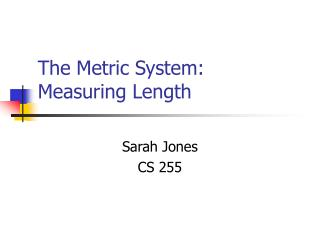DownloadDownload PresentationThe Metric System: Measuring Length

# The Metric System: Measuring Length

Télécharger la présentation## The Metric System: Measuring Length

- - - - - - - - - - - - - - - - - - - - - - - - - - - E N D - - - - - - - - - - - - - - - - - - - - - - - - - - -
##### Presentation Transcript

1. The Metric System: Measuring Length Sarah Jones CS 255

2. Introduction to the Metric System • The metric system first came about in the 1790’s when the French Academy of Science was asked to construct a new system of units for use throughout the world. • The metric system evolved over the years and in the 1960’s was named the International System of Measurement (SI for short). To get the meter, they divided a line from the north pole to the equator into 10 million parts!

3. No numbers to memorize Only 30 individual units Easy to say and write No fractions only decimals World standard Converts easily by multiples of 10. Advantages of Metric System

4. Prefixes and Multiplier: Kilo = 1000 Hecto = 100 Deca = 10 Meter = 1 Deci = .1 Centi = .01 Milli = .001 Examples: Kilometer Hectometer Decameter Meter Decimeter Centimeter Millimeter Units of Measurement

5. Length: • The standard unit of length in the metric system is the meter. Other units of length and their equivalents in meters are as follows: 1 millimeter = 0.001 meter1 centimeter = 0.01 meter1 decimeter = 0.1 meter1 decameter = 10 meters 1 hectometer = 100 meters 1 kilometer = 1000 meters

6. Abbreviations: • We abbreviate these lengths as follows:1 millimeter = 1 mm1 centimeter = 1 cm1 meter = 1 m1 decimeter = 1 dm1 kilometer = 1 km

7. Examples: • For reference, 1 meter is a little longer than 1 yard or 3 feet. It is about half the height of a very tall adult. A centimeter is nearly the diameter of a dime, a little less than half an inch. A millimeter is about the thickness of a dime.

8. Metric Conversion Steps For more on conversions visit:All you will need to know about metric.

9. 39,370 inches = 1 kilometer 3280.8 feet = 1 kilometer .62 miles = 1 kilometer 39 inches = 1 meter 3.28 feet = 1 meter 1 inch = 254 mm 1 inch = 2.54 cm 1 foot = 30.48 cm 1 yard = 91.44 cm 1 yard = .914 m 1 mile = 1609.3 m 1 mile = 1.609 km English to Metric Conversions You do not need to know these. They are just for you to get an idea of how these systems compare.

10. Metric Smile Project • ACTIVITY AND PROCEDURES: • Divide the class into groups of four. • Each student will measure and record the length of each person's smile in their group (in centimeters). • The students need to check their results against the results of the rest of the group. If there are any discrepancies the students should verify the results as a group.

11. Project (continued) • When an accurate measurement has been obtained for each child the results are recorded on the chalkboard as each child records them at their seat. • Order all the measurements from least to greatest. • Graph your results.

12. Last Steps • Find the sum of all the smiles in your classroom. Don't forget the teachers, it is often the largest and often forgotten because the students are so involved in this activity. • Create one smile out of construction paper that is the length of the of all the smiles in your room.